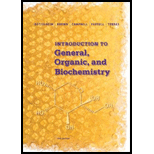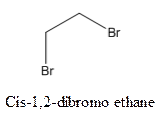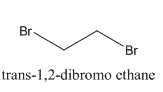# Draw a structural formula for at least one bromoalkene with the molecular formula C 5 H 9 Br that (a) shows cis/trans isomerism and (b) does not show cis /trans isomerism.### Introduction to General, Organic a...

11th Edition
Frederick A. Bettelheim + 4 others
Publisher: Cengage Learning
ISBN: 9781285869759### Introduction to General, Organic a...

11th Edition
Frederick A. Bettelheim + 4 others
Publisher: Cengage Learning
ISBN: 9781285869759

#### Solutions

Chapter
Section
Chapter 12, Problem 12.17P
Textbook Problem
237 views

## Draw a structural formula for at least one bromoalkene with the molecular formula C5H9Br that (a) shows cis/trans isomerism and (b) does not show cis /trans isomerism.

Expert Solution
Interpretation Introduction

Interpretation:

To draw a structural formula for at least one bromo-alkene with the molecular formula C5H9Br that show cis-trans isomerism.

Concept Introduction:

Isomers are the compounds that have same molecular formula but different structural formula.

Cis-trans isomerism arises in the compounds when there is a difference in the orientation of the two same groups.

When two same groups are along the same side of the C-C bond it is called as cis isomer, but when the same groups lie opposite to each other along the C-C bond. It is said to be trans isomer.

Example-andare cis-trans isomers.

### Explanation of Solution

The parent compound for C5H9Br is C5H10 that can have the skeleton as-

1. It can not have cis-isomers with molecular formula C5H9Br -

Expert Solution
Interpretation Introduction

Interpretation:

To draw a structural formula for at least Bromo-alkene with the molecular formula C5H9Br that does not show cis-trans isomerism.

Concept Introduction:

Isomers are the compounds that have same molecular formula but different structural formula.

Cis-trans isomerism arises in the compounds when there is a difference in the orientation of the two same groups.

When two same groups are along the same side of the C-C bond it is called as cis isomer, but when the same groups lie opposite to each other along the C-C bond. It is said to be trans isomer.

Example-andare cis-trans isomers.

### Want to see the full answer?

Check out a sample textbook solution.See solution

### Want to see this answer and more?

Bartleby provides explanations to thousands of textbook problems written by our experts, many with advanced degrees!

See solution